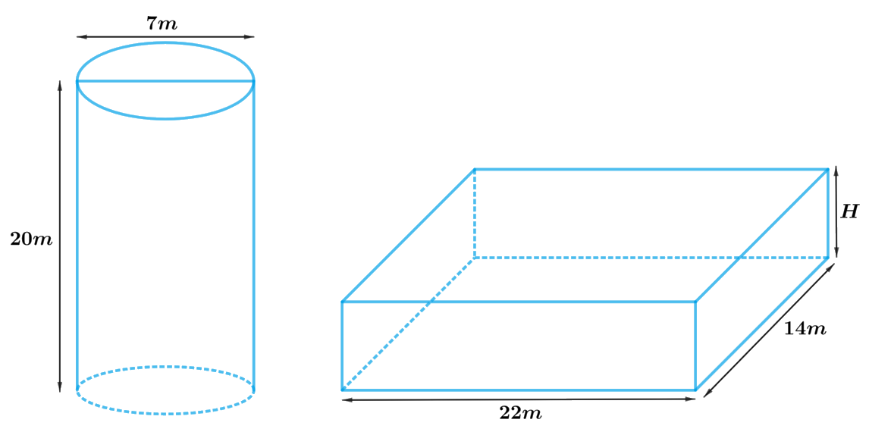# Ex.13.3 Q3 Surface Areas and Volumes Solution - NCERT Maths Class 10

Go back to  'Ex.13.3'

## Question

A $$20 \,\rm{m}$$ deep well with diameter $$7 \,\rm{m}$$ is dug and the earth from digging is evenly spread out to form a platform $$22\, \rm{m}$$ by $$14 \,\rm{m}$$. Find the height of the platform.

[Use \begin{align}\pi \;=\;\frac{22}{7}\end{align}]

Video Solution
Surface Areas And Volumes
Ex 13.3 | Question 3

## Text Solution

What is known?

Depth of the well is $$20\rm{m}$$ and diameter is $$7\rm{m}$$. Length of the platform is $$22\rm{m}$$ and breadth is $$14\rm{m.}$$

What is unknown?

The height of the platform.

Reasoning:

Draw a figure to visualize the shapes betterThe shape of the well will be cylindrical, and soil evenly spread out to form a platform will be in a cuboidal shape.

Therefore, volume of the soil dug from the well will be equal to the volume of soil evenly spread out to form a platform.

Volume of soil dug out from the well $$=$$ Volume of soil used to make such platform

Hence, Volume of the cylindrical well $$=$$ Volume of the cuboidal platform

We will find the volume of the cylinder and cuboid by using formulae;

Volume of the cylinder $$= \pi {r^2}h$$

where $$r$$ and $$h$$ are radius and height of the cylinder respectively

Volume of the cuboid $$= lbH$$

where $$l, b$$ and $$H$$ are length breadth and height of the cuboid respectively

Steps:

Depth of the cylindrical well,$$h = 20 \rm m$$

Radius of the cylindrical well,$$r = \frac{7}{2} \rm m$$

Length of the cuboidal platform,$$l = 22 \rm m$$

Breadth of the cuboidal platform,$$b = 14 \rm m$$

Let the height of the cuboidal platform $$= H$$

Volume of the cylindrical well $$=$$ Volume of the cuboidal platform

\begin{align}\pi {r^2}h &= lbH\\H &= \frac{{\pi {r^2}h}}{{lb}}\\&= \frac{{ \begin{bmatrix} \frac{{22}}{7} \times \frac{7}{2} \rm m \times\\ \frac{7}{2} \rm m \times 20 \rm m \end{bmatrix} }}{{22 \rm m \times 14m}}\\&= \frac{5}{2} \rm m\\&= \,2.5 \rm m\end{align}

Therefore, the height of such platform will be $$2.5 \rm{m}$$

Learn from the best math teachers and top your exams

• Live one on one classroom and doubt clearing
• Practice worksheets in and after class for conceptual clarity
• Personalized curriculum to keep up with school